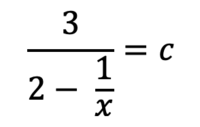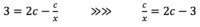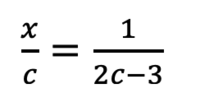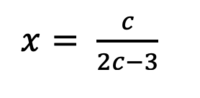# Taking the reciprocal of an equation

#### jpanknin

##### New member
Hi, everyone. I was given the following equation and asked to find all values of c such that the equation has no solutions for x.I worked through the following stepsThe book I'm using now says to take the reciprocal of both sides to get (this is the step I don't understand):and then multiply both sides by c to get:The part I don't understand is why take the reciprocal? Can anyone point me to a theorem or methodology or resource online that explains this approach/step in more detail?

#### pka

##### Elite Member
Hi, everyone. I was given the following equation and asked to find all values of c such that the equation has no solutions for x.

View attachment 26430
I worked through the following steps

View attachment 26436
The book I'm using now says to take the reciprocal of both sides to get (this is the step I don't understand):
View attachment 26434
and then multiply both sides by c to get:

View attachment 26437The part I don't understand is why take the reciprocal?
There is absolutely no reason to take the reciprocal of both sides.
From $$\dfrac{c}{x}=2c-3$$ it follows at once that $$x=\dfrac{c}{2c-3}$$ So $$c\ne\dfrac{3}{2}$$

#### Dr.Peterson

##### Elite Member
Hi, everyone. I was given the following equation and asked to find all values of c such that the equation has no solutions for x.
View attachment 26430
I worked through the following steps
View attachment 26436
The book I'm using now says to take the reciprocal of both sides to get (this is the step I don't understand):
View attachment 26434
and then multiply both sides by c to get:
View attachment 26437
The part I don't understand is why take the reciprocal? Can anyone point me to a theorem or methodology or resource online that explains this approach/step in more detail?
Are you asking why they chose to take the reciprocal, or why it is legal to do so? Those are two different questions.

Why do it? Because at that point they have x on the bottom, and what to get it out of the bottom. On the other hand, that is certainly not the only thing that can be done.

Why is it permitted? Because if a = b (and neither is zero), then 1/a = 1/b. That is obvious. I haven't seen many textbooks that explicitly state such a theorem, but in general you can apply any function to both sides of an equation, and this is just an example of that.

What else could be done instead? From c/x = 2c - 3, you can multiply both sides by x to get c = (2c - 3)x, and then divide to get x = c/(2c - 3). In the process, of course, it is required that x by nonzero, and that 2c - 3 be nonzero.

Or, you could start by simplifying the original equation by multiplying the numerator and denominator by x, giving 3x/(2x - 1) = c; then you can multiply by 2x - 1 to get 3x = 2xc - c, and solve for x to get x = c/(2c - 3).

So it is both legal and useful, but not necessary. Which of these three were you asking?

•JeffM

#### JeffM

##### Elite Member
To expand on Dr. Peterson’s answer, it is considered either an axiom or a theorem of arithmetic that

$$\displaystyle a,\ b, \text { and } c \in \mathbb R \text { and } a = b \implies ac = bc.$$

It is also an axiom or a theorem that

$$\displaystyle u \in \mathbb R \text { and } u \ne 0 \implies \dfrac{1}{u} \in \mathbb R \text { and } u * \dfrac{1}{u} = 1.$$

Now from those we can reason as follows.

$$\displaystyle \text {Given } p \in \mathbb R, \ p = q, \text { and } p \ne 0.$$

$$\displaystyle \therefore q \in \mathbb R \text { and } q \ne 0.$$

$$\displaystyle \dfrac{1}{p} \in \mathbb R \ \because \ p \in \mathbb R \text { and } p \ne 0.$$

$$\displaystyle \therefore p * \dfrac{1}{p} = q * \dfrac{1}{p} \ \because \ p = q \text { and } \dfrac{1}{p} \in \mathbb R.$$

$$\displaystyle \therefore 1 = \dfrac{1}{p} * q.$$

$$\displaystyle \dfrac{1}{p} * q \in \mathbb R \ \because \dfrac{1}{p} \text { and } q \in \mathbb R.$$

$$\displaystyle \dfrac{1}{q} \in \mathbb R \ \because \ q \in \mathbb R \text { and } q \ne 0.$$

$$\displaystyle \therefore 1 * \dfrac{1}{q} = \dfrac{1}{p} * \left ( q * \dfrac{1}{q} \right ).$$

$$\displaystyle \dfrac{1}{q} = \dfrac{1}{p} * 1 \implies \dfrac{1}{p} = \dfrac{1}{q}.$$

#### Jomo

##### Elite Member
2/3 = 2/3 AND 3/2 = 3/2

4/5 = 4/5 AND 5/4 = 5/4

1/x = 6/7 and x = 7/6 (one simple step)

When will this be untrue?

#### jpanknin

##### New member
Thanks @pka, @Jomo, @JeffM, and @Dr.Peterson. All very helpful. @Dr.Peterson, I think I was asking both of those questions. I initially got it by using the method from the fourth paragraph in your response (What else could be done instead?), but was unfamiliar with the book's approach of taking the reciprocal. Why it is permissible is now clear. Why they chose that method I guess is a matter of preference.

Appreciate all the responses very much.

•Jomo and Dr.Peterson